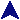Simple List OperationsProlog Examples```%
% Append lists: app(X,Y,Z) means that X append Y yields Z.  The base case says
% that anything appended to the empty list gives back the anything.  The
% second one says that, if T1 append X yeilds T2, then I can append A to the
% front of T1 and T2 and maintain the relationship.  More precisely, if T1
% append X yeilds T2, then [A|T1] append X yeilds [A|T2].
%
app([],X,X).
app([A|T1],X,[A|T2]) :- app(T1,X,T2).

%
% The length of the list: len(N, L) means N is the length of list L.  The
% basis rule says that the length of the empty list is 0.  The recursive
% rule says that, if N2 is the length of Tail, then N2 + 1 is the length of
% Tail with one additional member added to the front.  (Recall that _ is a
% variable.  The name _ is used when the value of a variable is not used
% elsewhere.)
%
len(0,[]).
len(N, [_ | Tail]) :- len(N2, Tail), N is N2 + 1.

%
% Take the sum of the members of a list of integers: sum_list(N, L) means that
% N is the sum of the members of L.  If SubTot is the sum of the members of
% Tail, then Head + SubTot is the sum of the members of [Head | Tail].
sum_list(0, []).
sum_list(Sum, [Head | Tail]) :- sum_list(SubTot, Tail), Sum is Head + SubTot.

%
% Reverse the list.
rev([],[]).
rev([H|T], R) :- rev(T, TR), app(TR, [H], R).

% Tell if the list (second argument) contains the first argument.
contains(X, [X|_]).
contains(X, [_|T]) :- contains(X,T).

```